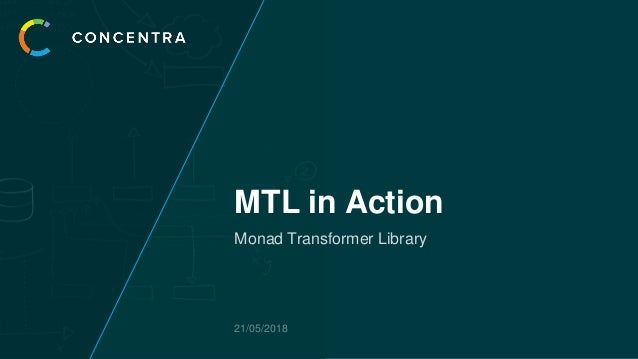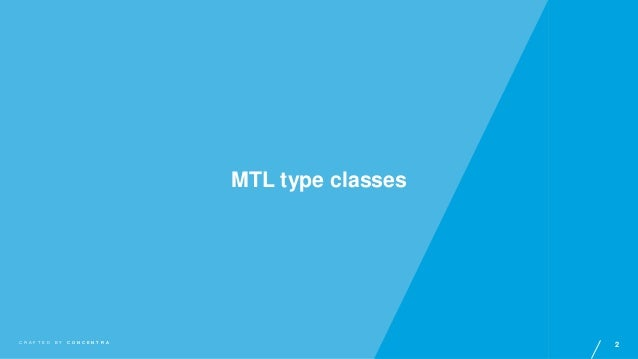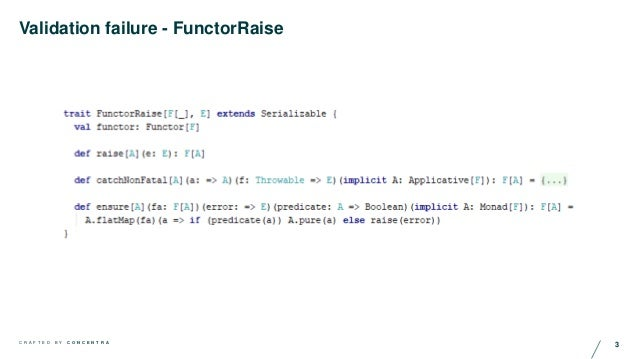Successfully reported this slideshow.Upcoming SlideShare
×

of0

Share

# Jamie Pullar- Cats MTL in action

At Concentra we have used abstract MTL patterns in our production service architecture for a number of years now. We are currently migrating across to the cats.mtl library which brings with it a heightened level of abstraction and composability whilst removing a lot of previously required boiler plate. In this talk I will give a brief overview of how to use cats mtl. Extol the benefits of implementing such an architecture. Share some of the more interesting consequences, as well as how we have resolved various challenges along the way.

See all

### Related Audiobooks

#### Free with a 30 day trial from Scribd

See all
• Be the first to like this

### Jamie Pullar- Cats MTL in action

1. 1. MTL in Action Monad Transformer Library 21/05/2018
2. 2. 2C R A F T E D B Y C O N C E N T R A MTL type classes
3. 3. 3C R A F T E D B Y C O N C E N T R A Validation failure - FunctorRaise
4. 4. 4C R A F T E D B Y C O N C E N T R A Validation failure - FunctorRaise
5. 5. 5C R A F T E D B Y C O N C E N T R A Events, Logs and Metrics - FunctorTell
6. 6. 6C R A F T E D B Y C O N C E N T R A Events, Logs and Metrics - FunctorTell
7. 7. 7C R A F T E D B Y C O N C E N T R A Interlude – Custom Ops
8. 8. 8C R A F T E D B Y C O N C E N T R A Environment or read-only state – ApplicativeAsk
9. 9. 9C R A F T E D B Y C O N C E N T R A Environment or read-only state – ApplicativeAsk
10. 10. 10C R A F T E D B Y C O N C E N T R A Read-write State – MonadState
11. 11. 11C R A F T E D B Y C O N C E N T R A Read-write State – MonadState
12. 12. 12C R A F T E D B Y C O N C E N T R A Chain it all together
13. 13. 13C R A F T E D B Y C O N C E N T R A Error Handling – ErrorMonad / ErrorApplicative class MyHandledService[M[_]: ApplicativeAsk[?[_], Connection]: MonadState[?[_], Map[UUID, String]]: FunctorTell[?[_], Vector[Event]]: ApplicativeError[?[_], NonEmptyList[String]]: Monad ] class MyCorrectlyHandledService[M[_]: ApplicativeAsk[?[_], Connection]: MonadState[?[_], Map[UUID, String]]: FunctorTell[?[_], Vector[Event]]: MonadError[?[_], NonEmptyList[String]] ]
14. 14. 14C R A F T E D B Y C O N C E N T R A Some historical context
15. 15. 15C R A F T E D B Y C O N C E N T R A May 2014 • Abstraction of Monad higher kinded type • Motivated by constant changing options for validation monads • Implemented via use of trait mixins • Lets pretend this never happened
16. 16. 16C R A F T E D B Y C O N C E N T R A May 2016 • Abstraction of Monad higher kinded type • Use of type classes for all monad types • Natural Transformations to move between stacks • Combinatorial boiler-plate nightmare • FutureT • Active in productive code for several years now • https://skillsmatter.com/skillscasts/8083-functional-service-oriented-architecture
17. 17. 17C R A F T E D B Y C O N C E N T R A May 2016 Reader Monad State Monad ~> ~> M[ _ ]:Error (ReaderStateError)
18. 18. 18C R A F T E D B Y C O N C E N T R A May 2018 • Abstraction of Monad higher kinded type • Use of type classes for all monad types • Use of composable cats MTL to create stacks • Service flow constructed around one Higher Kinded Type
19. 19. 19C R A F T E D B Y C O N C E N T R A May 2016 M[ _ ]:ErrorMonad M[ _ ]:ApplicativeAsk M[ _ ]:StateMonad M[ _ ]:ApplicativeAsk:StateMonad:ErrorMonad
20. 20. 20C R A F T E D B Y C O N C E N T R A May 2016
21. 21. 21C R A F T E D B Y C O N C E N T R A May 2016
22. 22. 22C R A F T E D B Y C O N C E N T R A May 2016
23. 23. 23C R A F T E D B Y C O N C E N T R A May 2016 trait KeyPersonRepo[M[_]] class KeyPersonStateRepo[M[_]:MonadState[?[_], Map[UUID, Person]]] extends KeyPersonRepo[M[_]] class KeyPersonSqlRepo[M[_]:ApplicativeAsk[?[_], Connection]] extends KeyPersonRepo[M[_]] type M[A] = ReaderT[Either[String, ?], Connection, A] UserCanAccess[M](new KeyPersonSqlRepo[M], new RoleAccessService[M])
24. 24. 24C R A F T E D B Y C O N C E N T R A Service Design
25. 25. 25C R A F T E D B Y C O N C E N T R A Monad Driven Service Design val result:Either[String, (Map[UUID, Person], Boolean)] = service.canUserAccess(uuid).run(state) • Returning an error will drop all writer logs and state changes • All ‘work’ is effectively annulled • This is not necessarily a bad thing but needs to be designed for • Unlifting errors out of the stack as explicit return type Have to reason about abstract dependencies however • Can change behaviour but does change how we might reason on our program
26. 26. 26C R A F T E D B Y C O N C E N T R A Monad Driven Service Design • If only a small section of the service requires a given mtl function, can you abstract out to a dependency? • Simplifies testing • Primarily consider extraction for Reader, State, and IO Minimise mtl requirements
27. 27. 27C R A F T E D B Y C O N C E N T R A Monad Driven Service Design State and Environment Products MonadState[M, Map[String, String]] MonadState[M, Map[String, Int]]+ MonadState[M, (Map[String, String], Map[String, Int])]
28. 28. 28C R A F T E D B Y C O N C E N T R A Monad Driven Service Design State and Environment Products def tupleStateMonad[M[_], S <: Product, S2](implicit S:Selector[S, S2], R:Replacer[S, S2, S2], M:MonadState[M, S]):MonadState[M, S2] = new MonadState[M, S2] { val monad: Monad[M] = M.monad def inspect[A](f: S2 => A):M[A] = M.inspect(s => f(S(s))) def modify(f: S2 => S2):M[Unit] = M.modify(s => R(s, f(S(s))).asInstanceOf[(S2, S)]._2) def get:M[S2] = M.inspect(S.apply) def set(s2: S2): M[Unit] = M.modify(s => R(s, s2).asInstanceOf[(S2, S)]._2) }
29. 29. 29C R A F T E D B Y C O N C E N T R A Monad Driven Service Design State and Environment Products def tupleApplicativeAsk[M[_], E <: Product2[_,_], E2](implicit S:Selector[E, E2], A:ApplicativeAsk[M, E]):ApplicativeAsk[M, E2] = new ApplicativeAsk[M, E2] { val applicative: Applicative[M] = A.applicative def ask:M[E2] = A.reader(S.apply) def reader[A](f: E2 => A):M[A] = A.reader(e => f(S(e))) }
30. 30. 30C R A F T E D B Y C O N C E N T R A Monad Driven Service Design State and Environment Products implicit def mInt:MonadState[M, Map[String, Int]] = tupleStateMonad[M, (Map[String, String], Map[String, Int]), Map[String, Int]] implicit def mString:MonadState[M, Map[String, String]] = tupleStateMonad[M, (Map[String, String], Map[String, Int]), Map[String, String]] https://stackoverflow.com/questions/50271244/avoid-diverging-implicit-expansion-on-recursive-mtl-class
31. 31. 31C R A F T E D B Y C O N C E N T R A Monad Driven Service Design Service Provider Pattern class UserAnalytics[M[_]](userServiceProvider:TenantId => M[UserService[M[_]]]) { def getUserCountForTenant(tenantId:TenantId):M[Int] = { for { userService <- userServiceProvider(tenantId) count <- userService.count } yield count } } class TestUserService[M[_]:State[Map[UUID, User], ?]] type M[A] = State[(Map[TenantId, Map[UUID, User]], Map[UUID, User]), A]
32. 32. 32C R A F T E D B Y C O N C E N T R A Performance
33. 33. 33C R A F T E D B Y C O N C E N T R A Performance Simple benchmark 1000x class Baseline { def run = { val a = "a" val b = "b" val ab = a + b val c = "c" val abc = ab + c val d = "d" val dc = d + c val e = "e" val f = "f" val g = "g" val efg = e + f + g val h = "h" val afh = a + f + h val i = "i" val j = "j" val k = "k" val l = "l" val ijkl = i + j + k + l val dijkl = d + ijkl val m = "m" val n = "n" val mn = m + n val o ="o" ab + dc + afh + ijkl + mn + o } } class MapFlatMapService[M[_]:Monad] { def run:M[String] = for { a <- pure("a") b <- pure("b") ab = a + b c <- pure("c") abc = ab + c d <- pure("d") dc = d + c e <- pure("e") f <- pure("f") g <- pure("g") efg = e + f + g h <- pure("h") afh = a + f + h i <- pure("i") j <- pure("j") k <- pure("k") l <- pure("l") ijkl = i + j + k + l dijkl = d + ijkl m <- pure("m") n <- pure("n") mn = m + n o <- pure("o") } yield ab + dc + afh + ijkl + mn + o }
34. 34. 34C R A F T E D B Y C O N C E N T R A Performance Monads 0 0.1 0.2 0.3 0.4 0.5 0.6 0.7 0.8 0.9 Either Monad Id Monad Baseline Time(ms)
35. 35. 35C R A F T E D B Y C O N C E N T R A Performance Monad Transformer Stack 0 1 2 3 4 5 6 7 8 9 ReaderTStateTWriterTEither Either Monad Id Monad Baseline Time(ms) type M[R, S, L, E, A] = ReaderT[StateT[WriterT[Either[E, ?], L, ?], S, ?], R, A]
36. 36. 36C R A F T E D B Y C O N C E N T R A Performance ReaderWriterState Monad 0 1 2 3 4 5 6 7 8 9 ReaderWriterState ReaderTStateTWriterTEither Either Monad Id Monad Baseline Time(ms) final class IndexedReaderWriterStateT[F[_], E, L, SA, SB, A](val runF: F[(E, SA) => F[(L, SB, A)]]) extends Serializable { type ReaderWriterState[E, L, S, A] = ReaderWriterStateT[Eval, E, L, S, A]
37. 37. 37C R A F T E D B Y C O N C E N T R A Performance ReaderWriterStateTEither final class IndexedReaderWriterStateT[F[_], E, L, SA, SB, A](val runF: F[(E, SA) => F[(L, SB, A)]]) extends Serializable { type ReaderWriterStateTEither[E, L, S, A] = ReaderWriterStateT[Either[V, ?] , E, L, S, A] 0 1 2 3 4 5 6 7 8 9 ReaderWriterStateTEither ReaderWriterState ReaderTStateTWriterTEither Either Monad Id Monad Baseline Time(ms)
38. 38. 38C R A F T E D B Y C O N C E N T R A Performance Service Monad 0 1 2 3 4 5 6 7 8 9 ServiceMonad ReaderWriterStateTEither ReaderWriterState ReaderTStateTWriterTEither Either Monad Id Monad Baseline Time(ms) final case class ServiceMonad[R, S, L, E, T](f:(R, S) => Either[E, (S, L, T)]) {
39. 39. 39C R A F T E D B Y C O N C E N T R A Performance Unstacked Monad 0 1 2 3 4 5 6 7 8 9 Unstacked Monad ServiceMonad ReaderWriterStateTEither ReaderWriterState ReaderTStateTWriterTEither Either Monad Id Monad Baseline Time(ms)
40. 40. 40C R A F T E D B Y C O N C E N T R A Performance Unstacked Monad sealed trait UnstackedMonad[R, S, L, E, A] final case class UnstackedError[R, S, L, E, A](e:E) extends UnstackedMonad[R, S, L, E, A] final case class UnstackedPure[R, S, L, E, A](a:A) extends UnstackedMonad[R, S, L, E, A] final case class UnstackedWriter[R, S, L, E, A](l:L, a:A) extends UnstackedMonad[R, S, L, E, A] final case class SuccessReturn[S, L, A] private(s:S, l:L, a:A) final case class ErrorReturn[E] private(e:E) final case class UnstackedFunction[R, S, L, E, A](func:(R, S) => Any) extends UnstackedMonad[R, S, L, E, A]
41. 41. 41C R A F T E D B Y C O N C E N T R A Performance Unstacked Monad final case class UnstackedError[R, S, L, E, A](e:E) extends UnstackedMonad[R, S, L, E, A] final case class UnstackedErrorWithLog[R, S, L, E, A](l: L, e:E) extends UnstackedMonad[R, S, L, E, A] final case class UnstackedErrorWithLogAndState[R, S, L, E, A](s:S, l:L, e:E) extends UnstackedMonad[R, S, L, E, A]
42. 42. 42C R A F T E D B Y C O N C E N T R A We need to talk about Futures
43. 43. 43C R A F T E D B Y C O N C E N T R A Supporting futures trait LiftFuture[M[_]] { def liftFuture[A](f: => Future[A]):M[A] } private def requestWithMethod( method: HttpMethod, url: String, headers:List[HttpHeader], contentType: ContentType, content: Array[Byte]): M[Array[Byte]] = for { res <- liftFuture { Http().singleRequest(HttpRequest(method, Uri(url), headers, HttpEntity(contentType, content))) } r <- status(url, res) d <- liftFuture { r.entity.dataBytes.runFold(ByteString(""))(_ ++ _).map(e => e.toIterator.toArray) } } yield d
44. 44. 44C R A F T E D B Y C O N C E N T R A Supporting futures final case class UnstackedFuture[R, S, L, E, A](func:(R, S) => Any) extends UnstackedAsyncMonad[R, S, L, E, A] final case class SuccessReturn[S, L, A] private(s:S, l:L, a:A) final case class ErrorReturn[E] private(e:E) final case class FutureReturn private(f:Future[Any])
45. 45. 45C R A F T E D B Y C O N C E N T R A Supporting futures class EffectfulActorServiceWrapper[D[_], M[_], N[_]:LiftFuture:Monad] (service: D ~> M, effect: => Effect[M, N], name:Option[String]) (implicit af:ActorRefFactory, timeout:Timeout) extends (D ~> N) { import akka.pattern._ val e:Effect[M, N] = effect def props = Props { new Actor { def receive: PartialFunction[Any, Unit] = { case d: D[_]@unchecked => sender ! e.unsafeRun(service(d)) } } } val actorRef: ActorRef = name.fold(af.actorOf(props)){ n => af.actorOf(props, n)} def apply[A](fa: D[A]):N[A] = implicitly[Monad[N]].flatten(implicitly[LiftFuture[N]].liftFuture(actorRef.ask(fa).asInstanceOf[Future[N[A]]])) }
46. 46. GET IN TOUCH • GET THE EDGE Concentra Analytics 100 Cheapside London EC2V 6DT +44 (0)20 7099 6910 info@concentra.co.uk concentra.co.uk

Total views

984

On Slideshare

0

From embeds

0

Number of embeds

1

11

Shares

0